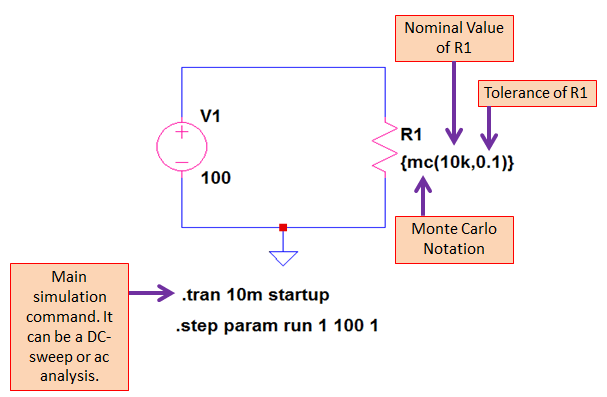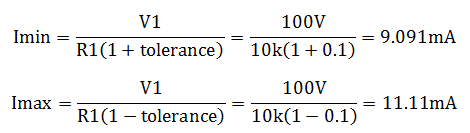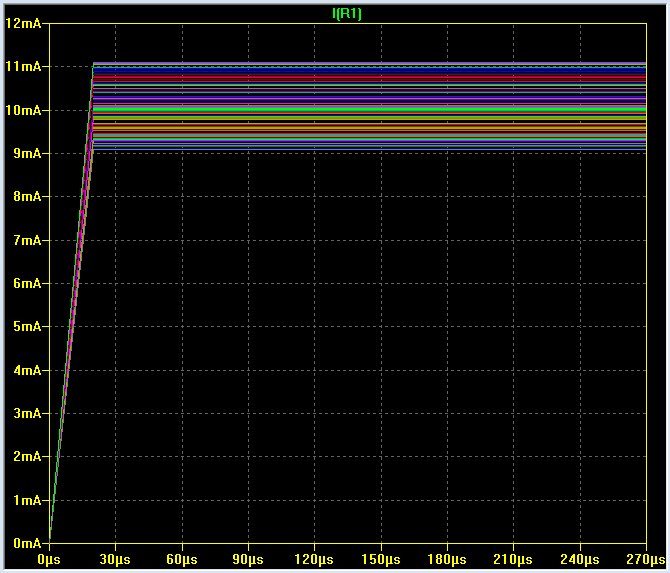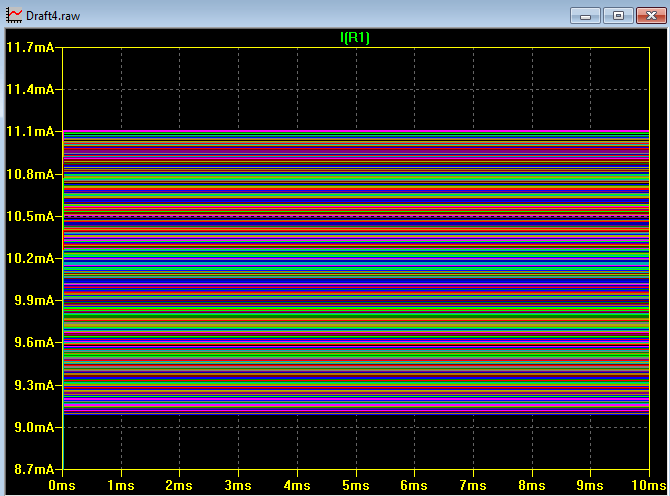# Worst Case Analysis and Tolerance Simulation Using LTSpice

I believe there are few ways to perform worst case analysis and tolerance simulation using LTSpice. In this article, I will be sharing my own technique. This can be an easier approach and at the same time you will have your Monte Carlo analysis and simulations already. Basically, I will be making use of the Monte Carlo run to come up with the worst case circuit parameter.

If we talk about worst case, it is the extremes of the design or a circuit. It can be the minimum and maximum current, minimum and maximum voltage or minimum and maximum power dissipation. It therefore involves the circuit tolerances.

To formally start this worst case analysis and tolerance simulation using LTSpice, let us consider a very simple circuit below; a voltage source and a resistor.Supposing these are the circuit given,

V1 = 100V and R1 = 10kΩ, 10% tolerance

Based on calculations, the worst case currents will beNow, let us do simulation and our objective is to match the result to the computed min and max values above.

This will be our circuit modelIf you read my post on Monte Carlo Simulations using LTSpice with Step by Step Tutorials, you can see the same syntax I am using here. Yes it is.

In this particular worst case analysis and tolerance simulation using LTSpice, we are going to extract the minimum and maximum values out of the Monte Carlo run.

In the above syntax, the variable “mc” specifies a Monte Carlo analysis on the 10k ohms resistor with a 10% tolerance. To make the Monte Carlo run successfully, a .step command must be executed. In below .step command, the simulation is run up to 100 times with a starting count of 1 and increment of 1.Let us run the simulation. Our main simulation command is transient. So we can expect to see a startup scenario on the waveforms.

Below is the simulation result for the circuit current. It contains many current values. Where are the worst case currents? Simple, the highest current on the waveform is the maximum current, while the lowest current is the minimum current.Let us find out if indeed we get the same values with the computations. Based on my measurement, we got below min and max values.We got very close min and max values with respect to the computations. By making the number of run many times, the exact worst case values you can get. For simple circuit like we did, 100 run is enough to get the worst case. For circuits with many tolerances involve, increase the number of runs. In our circuit model, if I increase the number of run, there will be more effect to the min and max values. Below is the result when I increase the number of run to 1000.## Worst Case Analysis and Tolerance Simulation Using LTSpice Conclusion

Worst case analysis is can be done at the same time with a Monte Carlo simulation in LTSpice. The minimum and maximum level displayed in the waveform is the worst case values for a particular parameter.

However, the result is not accurate if there is only few number of run. To make it accurate, simply increase the number of run. For a very simple circuit with one tolerance involve will only need 100 runs to get the accurate minimum and maximum values. For circuits with several tolerances, a good result will be observed from 700-1000 runs. Try this with yourself and find out if this thing can help.

## One comment

1. Dave Blanchard says:

I tried your technique to do a monte carlo simulation, however, when I tried to run the program an error message is displayed indicating it can not find definition of model MC for each component using the form {mc(value,tolx)}. How does one get by this error?

This site uses Akismet to reduce spam. Learn how your comment data is processed.